Journal of Global Change Data & Discovery2019.3(1):19-26

Citation：Bi, S. W., Rao, S. W.A Segmentation Algorithm for Quantum Remote Sensing Image Data[J]. Journal of Global Change Data & Discovery,2019.3(1):19-26 .DOI: 10.3974/geodp.2019.01.03 .

# A Segmentation Algorithm for Quantum Remote Sensing Image Data

## Bi, S. W.*  Rao, S. W.

State Key Laboratory of Remote Sensing Science, Institute of Remote Sensing and Digital Earth, Chinese Academy of Sciences, Beijing 100101, China

Abstract: Quantum remote sensing imaging is a hot topic in the field of the updated remote sensing image data processing. The remote sensing image segmentation is one of the most useful methods among them. Developing a segmentation algorithm in remote sensing image data processing can be based on the concept of quantum theory. The segmentation method proposed in this study is called Bi’s quantum segmentation method. The quantum genetic algorithm used in this method can automatically perform a multi-threshold search on the remote sensing image to be segmented, and its search efficiency was verified through simulation experiments. Compared with the traditional method, the segmentation method proposed here can more effectively retain information from the original image, and significantly improve the accuracy and stability of multi- threshold image segmentation results. Bi’s quantum segmentation method is a new technique that will facilitate growth in the segmentation processing of remote sensing image data.

Keywords: quantum remote sensing; image segmentation; quantum genetic algorithm; maximum entropy; optimal threshold

### 1 Introduction

Since Prof. Bi, Siwen proposed the concept of quantum remote sensing (QRS), they go through several study stages, including QRS theory, QRS information, QRS experiments, QRS imaging, QRS calculation, and QRS measurements. For the past ten years, the author and his nine graduate students have successfully studied the QRS image data denoising and enhancement algorithms, which has laid the foundation for the following studies on quantum image processing (such as edge extraction and image segmentation).

Remote sensing image data segmentation is a process in which remote sensing (RS) images are sub-divided into uncrossed sub-regions where the information has the same characteristics, such as its grayscale values, textures, colors, and contrast features. Image segmentation technology can effectively simplify or change the expression of an image and enable the extraction of targets of interest from the image, making the image easier to be understood and analyzed. Image segmentation methods mainly include the threshold, edge-based, and region-based methods. In this study, we used the threshold method to perform the image segmentation.

The threshold image segmentation method can be either a single-threshold or multi- threshold process. The former is used to determine an optimal threshold for image segmentation, and thus achieve the image binarization. This is simple and effective, particular effective for images with obvious bi-modal gray histograms. However, most images, especially RS images, have complex textures and blurred boundaries with large amounts of information. Therefore, the required image targets are not clear. Such image histograms have multiple peaks and undulations. Under this condition, single-threshold image segmentation can not reflect the image characteristics, and multi-threshold segmentation is necessary for subsequent processing. The rapid and efficient determination of the optimal segmentation threshold is the key to the multi-threshold image segmentation method.

Common threshold methods include the OTSU algorithm, fuzzy K-means algorithm, two-peak method, and K-means algorithms. In recent years, the image threshold method based on the maximum entropy and maximum fuzzy entropy has achieved better results than the traditional methods, and therefore has attracted much attention. Pun first proposed the image threshold segmentation method based on maximum entropy criterion. Kapur et al. further refined this approach. Subsequently, Cheng et al. combined the fuzzy mathematics theory with the maximum entropy and proposed the maximum fuzzy entropy image segmentation method. Based on this, Xu proposed an improved multi-threshold image segmentation method and achieved a better effect. These image segmentation methods, based on maximum entropy criterion, often use the full-search method to determine the optimal multi-threshold combination. Although this method can obtain a global optimal solution, its application is limited due to the complex programming, and time-costing and computing requirements.

The quantum genetic algorithm (QGA) is a quantum intelligent algorithm that combines quantum mechanics theory with the conventional genetic algorithm (CGA)[15–19]. QGA introduces the state vector representation in sub-mechanics into chromosome coding. In the evolution mechanism, a quantum revolving gate is used to realize chromosome evolution. The quantum properties help the QGA to obtain a better population diversity, faster convergence speed and stronger global search ability than traditional genetic algorithms. This new and improved algorithm has a far superior performance to traditional algorithms. Therefore, this study combined the maximum entropy criterion and QGA, to develop a multi-threshold segmentation method based on the gray image of the QGA. Results showed that the proposed algorithm improved the reliability and stability of image segmentation and can obtain better segmentation results compared to traditional algorithms.

### 2 The Quantum Segmentation Algorithm

Both the QRS image denoising and QRS image enhancement use the pixel qubit form mentioned in references . The general principle is that supposing g(m, n) is the original image, with a resolution of M´N, and f(m, n) is its normalized image, which can be represented as a two-dimensional matrix of M´N: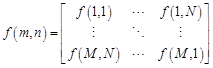(1)

The right side of the expression defines a digital image. Each element in the matrix is an image pixel. Image normalization transformation is generally: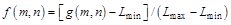(2)

where Lmax and Lmin represent the maximum and minimum gray values of the original image, respectively, and f(m, n)[0,1], represents the normalized gray value of pixel g(m, n). To realize the mapping of images from gray space to quantum space, the following pixel qubit expression was defined by referring to the probability statistics in reference :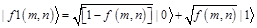(3)

where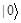and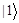express the state of the black and white points, respectively. The probability amplitudes of their corresponding ground states are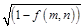and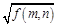which satisfy the normalized condition.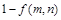and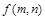represent the appearance probability of white and black pixels, respectively.

When g(m, n) = 0.5×(Lmax+Lmin), the appearance probabilities of white and black states are the same, while white and black, and light and dark are relative concepts. Even for different images with the same maximum and minimum gray values, the distribution of gray histograms will not be exactly the same. Similarly, image segmentation also uses the principle of quantum superposition states, and its specific definition is as follows.

2.1 Quantum Chromosome Coding

In genetic algorithms, chromosomes are represented by certain values. Common coding methods include binary, decimal, and symbolic, etc. The chromosomes of the QGA are represented by random probability qubit encoding. A quantum chromosome with m qubit genes can be described as: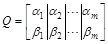(4)

where |αi|2+|βi|2=1, the ith qubit gene can be defined as [αi, βi], i = 1, 2, .., m by probability amplitude, and such an expression can express any quantum superposition. For example, a three-qubit system (the first line means the probability amplitude of “0”, and the second means the probability amplitude of “1”):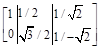(5)

Then its sub-system can be expressed as: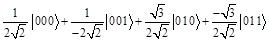(6)

The above results show that the appearance probability of the four ground states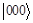,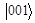,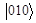, and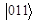in the system are 1/8, 1/8, 3/8 and 3/8, respectively, and therefore the quantum subsystem of Equation (6) can represent four kinds of state information simultaneously. A quantum chromosome no longer expresses a certain piece of information, but contains all of the possible information, and any operation on the gene in the chromosome will act on all possible states simultaneously. This ensures that the QGA has a better diversity than the CGA and the QGA population size is much smaller than that of the CGA for the same optimization problem. In addition, a better convergence can be obtained by using quantum chromosome coding. As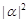or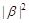tends to 0 or 1, the qubit-encoded chromosome will converge to a certain state.

2.2 Quantum Crossing

Crossing is a means that genetic algorithm mimics the genetic recombination of sexual reproduction in nature, enabling the inheritance of superior genes from the previous generation to new individuals and evolved with more complex genetic structures, which can avoid the precocity phenomenon to a certain extent. In CGA, crossing includes single-point crossing, multiple-point crossing, and consistent crossing.

2.3 Quantum Variation

During biological genetics and evolution, some genes in DNA chromosomes may be mutated due to accidental or specific factors during cell division and replication. They will have new biological traits and produce new chromosomes. Mutation refers to the change of one or several genes in a chromosome coding sequence to generate new individuals. Quantum mutation is an effective means to improve the performance of the QGA in searching for an optimal solution. In quantum mechanics theory, the transformation between states is achieved through quantum gates. Common quantum gate transformation matrices include revolving gates, XOR gates, NOT gates, and Hadamard gates. The mutation of a quantum chromosome can also be realized through a quantum gate, using the information of optimal individual to guide chromosome evolution in the population and improve the convergence of the QGA. A common quantum rotation gate is defined as follows: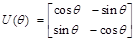(7)

where q indicates the rotational variation angle of the quantum rotation gate, whose size and direction are based on a pre-designed adjustment strategy. The quantum rotation variation operation is: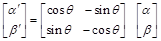(8)

where [α β]′ is a qubit vector of the gene. The operation of quantum rotation mutation is to achieve the transition among states and get the algorithm converge.

2.4 Multi-threshold Segmentation Algorithm Flow Using Maximum Entropy and the QGA

The process is as follows:

(1) Initialize evolutionary algebra at t=0 and population Q(t);

(2) Collect statistics regarding image data information entropy;

(3) Calculate the best fitness value of the function and the corresponding entropy to obtain the mean value;

(4) At t=t+1, update the population through quantum mutation and quantum rotation gates;

(5) If the mean entropy reaches maximum, output the best result, otherwise return to step 2).

2.5 Quantum Image Data Segmentation Algorithm Flow Chart

The flow chart of image data segmentation is shown in Figure 1, including initializing population, collecting image data information entropy, individual measurement, population regeneration, and output of the best individual.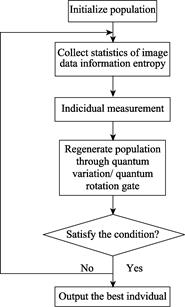Figure 1  Flow chart of image data segmentation using maximum entropy and the quantum genetic algorithm

### 3 Simulation Experiment and the Results of the Quantum Image Data Segmentation Algorithm

A simulation experiment was conducted on the algorithm using C++ and the openCV library in the Visual Studio 2013 integrated development environment. Segmentation image results (qualitative), data statistics (quantitative), and the average running time (each method was tested for 10 times) were compared for the OTSU (maximum inter-class variance) algorithm, K-means clustering algorithm, maximum entropy threshold segmentation method and the quantum segmentation method. An image segmentation experiment was conducted on a computerized tomography (CT) scan of a patient’s pathological heart and an aerial image of an airport obtained by RS. The results are shown below.

The results showed that the quantum segmentation algorithm had a better segmentation effect than the traditional methods, such as the OTSU, K-means and maximum entropy threshold algorithms. Segmented images had a clearer texture and structure for both the CT of a patient’s pathological heart (Figure 2) and the aerial image of an airport (Figure 3). More importantly, as shown in Tables 1 and 2, the information entropy of the quantum segmentation algorithm was higher (>0.1) than that of the best traditional methods while the running time was almost the same, proving that the quantum method can obtain better segmentation results. Images segmented by this method retained more information from the original image than images segmented using the traditional methods.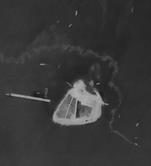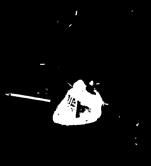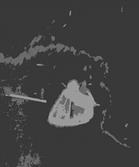(a) Original image                       (b) OTSU algorithm              (c) K-means algorithm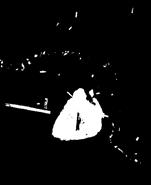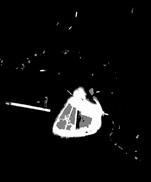(d) Maximum entropy threshold segmentation method (e) Bi’s quantum segmentation method

Figure 2  Image results for four different segmentation algorithms

Table 1  Comparison of the segmentation results for the four different methods

 Segmentation algorithm Image information entropy after segmentation Average running time (s) OTSU algorithm 0.581,895 0.052,065 K-means algorithm 0.553,726 0.048,037 Maximum entropy threshold   segmentation method 0.727,849 0.062,642 Bi’s quantum segmentation   method 0.921,277 0.832,738

### 4 Conclusion

Based on the basic principle of maximum entropy image segmentation, a multi-threshold image segmentation method based on adaptive maximum entropy has been developed. To improve the multi-threshold solving efficiency, this study applied QGA as an RS image segmentation method to determine the optimal threshold combination. This reduced the complexity of programming and improved the accuracy and robustness of multi-threshold image segmentation results. To avoid generating invalid multi-threshold solutions in the improved QGA, the study used an efficient coding method for binary value solutions, and then defined an adaptive rotation angle when using quantum rotation gates. The results showed that the multi-threshold image segmentation method could better segment the target in an image than the traditional segmentation algorithm and more image information was retained for the same number of segmentation thresholds.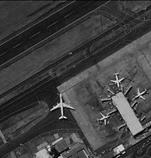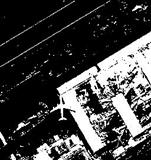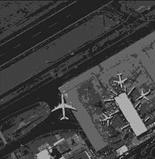(a) Original image                   (b) OTSU algorithm              (c) K-means algorithm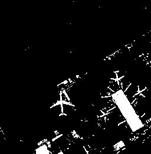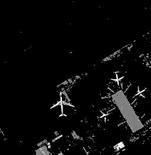(d) Maximum entropy threshold segmentation method (e) Bi’s quantum segmentation method

Figure 3  Image results for four different segmentation algorithms

Table 2  Comparison of the segmentation results for the four different methods

 Segmentation algorithm Image information entropy after segmentation Average running time (s) OTSU algorithm 0.793,13 0.066,112 K-means algorithm 0.989,53 0.042,504 Maximum entropy threshold   segmentation method 1.234,58 0.056,347 Bi’s quantum segmentation method 1.518,22 0.827,539

### 5 Prospect

The Quantum remote sensing imaging is a hot topic in the field of remote sensing image data processing. The proposed Bi’s quantum segmentation method may enable the field of remote sensing image data segmentation to develop in new directions. In this study, the adaptive multi-threshold search algorithm involved in segmenting remote sensing images improved the automatic search efficiency of the image segmentation multi-threshold, and improved the accuracy and stability of multi-threshold image segmentation results. In image processing, there are still many problems that need to be globally optimized, such as the determination of the optimal threshold in wavelet denoising and the determination of morphological structural elements. It may be possible to apply a QGA to a related image processing method. However, it should be noted that in the existing QGA, the selection of the number of thresholds and the number of quantum gene positions is artificially set, and other methods can be used to determine their optimal values. In addition, chromosome updates and mutations are usually implemented using quantum revolving gates. Other quantum gates in quantum computing can also be considered to use in evolutionary algorithms, which may further improve the overall optimization efficiency and performance of the algorithm.

### References

       Bi, S. W. Research on the concept, framework and connotation of quantum remote sensing [J]. Journal of Infrared and Millimeter Waves, 2003, 22: 19.

       Bi, S. W., Han, J. X. Study of Information mechanism of quantum remote sensing [J]. Science & Technology Review, 2006, 24(9): 3842.

       Bi, S. W., Han, J. X. Experimental study of mid and far-infrared in quantum remote sensing [C]. Proceedings of the 15th National Remote Sensing Conference, 2005.

       Chen, L., Bi, S. W., Lu, B. Z. Experimental study on the imaging of the squeezed state light at 1064 nm, Laser Physics, 2011, 21(7): 12021207.

       Bi, S. W. Calculating time and time retrieval operators of quantum remote sensing [J]. Journal of Infrared and Millimeter Waves, 2003, 22(Sup.): 7075.

       Bi, S. W., Chen, H. A denoising algorithm for quantum remote sensing image data [J]. Journal of Global Change Data & Discovery, 2018, 2(3): 256270. DOI: 10.3974/geodp.2018.03.03.

       Bi, S. W., Ke, Y. X. Enhancement algorithm for quantum remote sensing image data [J]. Journal of Global Change Data & Discovery, 2018, 2(4): 367376. DOI: 10.3974/geodp.2018.04.01.

       Xu, E. J. Remote sensing image segmentation based on K-means [D]. Urumqi: Xinjiang University, 2014.

       Chen, M., Wang, X. F. Multi-threshold remote sensing image segmentation based on simulated annealing [J]. Information Systems Engineering, 2011, 12(8): 7375.

    Yang, K., Jiang, H. W. Research on improved algorithm for multilevel thresholding image segmentation based on fuzzy maximum entropy [J]. Computer Engineering and Applications, 2009, 45(32): 174177.

    Pun, T. A new method for grey-level picture thresholding using the entropy of the histogram [J]. Signal Processing, 1980, 2(3): 223237.

    Pun, T. Entropic thresholding, a new approach [J]. Computer Graphics and Image Processing, 1981, 16(3): 210239.

    Kapur, J., Sahoo, P., Wong, A. A new method for gray-level picture thresholding using the entropy of the histogram [J]. Computer vision, graphics and image processing, 1985, 29(3): 273285.

    Cheng, H., Chen, J., Li, J. Threshold selection based on fuzzy C-partition entropy approach [J]. Pattern Recognition, 1998, 31(7): 857870.

    Han, K. J. Genetic quantum algorithm and its application to combinatorial optimization problem [C]. Proceedings of IEEE International Conference on Evolutionary Computation, 2000: 13541360.

    Han, K. J. Quantum-inspired evolutionary algorithm for a class of combinatorial optimization [J]. IEEE Transactions on Evolutionary Computation, 2002, 6(6): 580593.

    Han, K., Kim, J. On setting the parameters of quantum-inspired evolutionary algorithm for practical applications. In: Congress on Evolutionary Computation. Canberra, Australia: Citeseer, 2003: 178184.

    Han, K., Kim, J. Quantum-inspired evolutionary algorithms with a new termination criterion, He Gate, and two-phase scheme [J]. IEEE Transactions on Evolutionary Computation, 2004, 8(2): 156169.

    Han, K., Park, K., Lee, C., et al. Parallel quantum-inspired genetic algorithm for combinatorial optimization problem [C]. Proceedings of the 2001 IEEE Congress on Evolutionary Computation, 2001: 14221429.

    Tseng, C. C., Tsung, M. Quantum digital image processing algorithms [C]. In: 16th IPPR Conference on Computer Vision, Graphics and Image Processing (CVGIP 2003), Kinmen, 2003: 827834.

    Xu, W. S. Research on digital image processing based on quantum theory [D]. Changsha: Hunan Normal University, 2013.

    Fu, X. W. Research on image processing methods based on quantum mechanics [D]. Wuhan: Huazhong University of Science and Technology, 2010.

Networking in Top